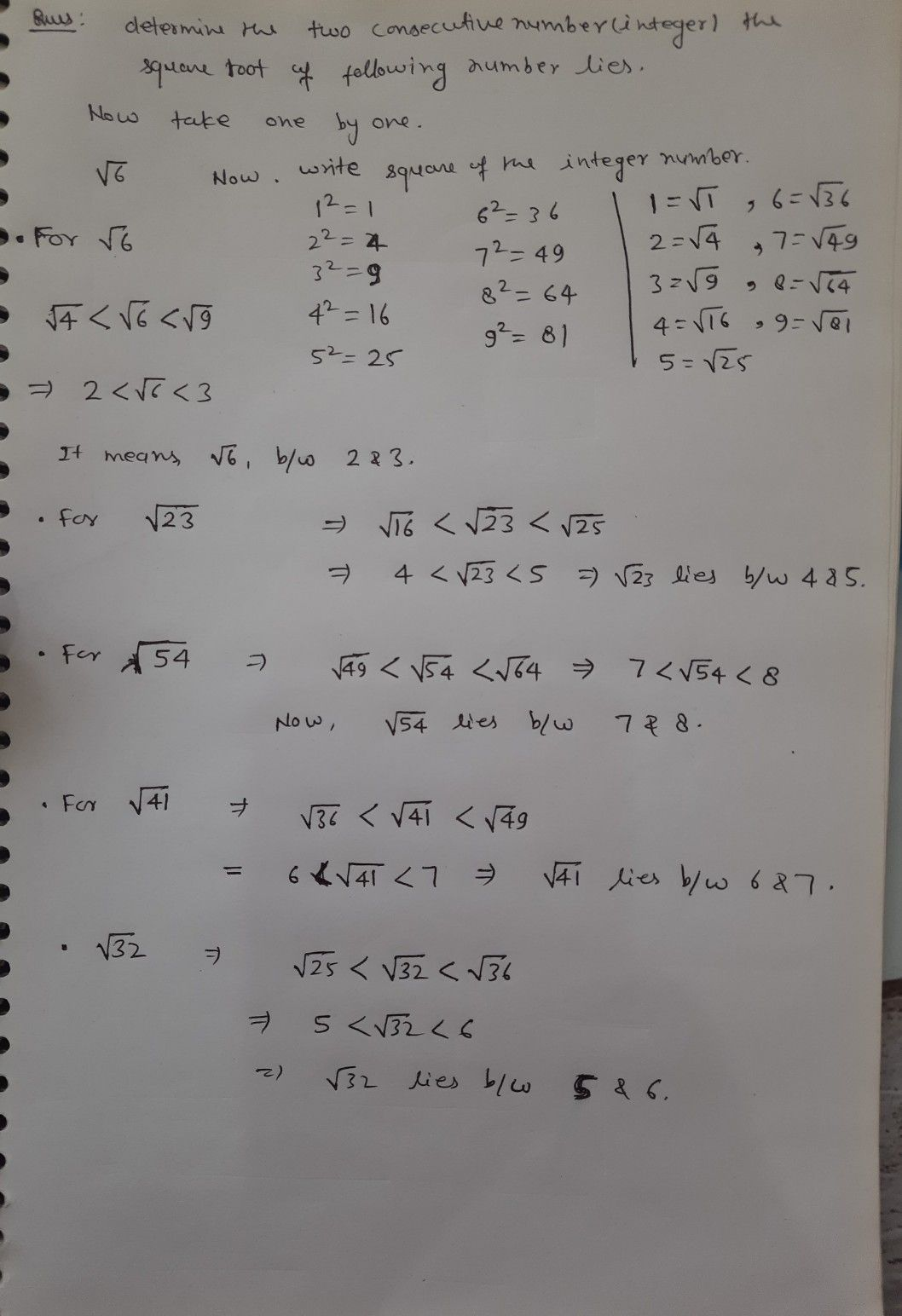Symbol
Problemm this module, you will learn hovw to estimats the sauare root of a mmber to the nearest $21c11$ and plots arational numtvers (up to square roots) on a umber line What's $Im$ Determine between what two conecutive integers the square root of he $11°1o11o$ $nngtoaimbs$ $5$ $\sqrt{32}$ 2. VE3 3 $\bar{51}$ $4\sqrt{41}$ What's New Can you give the square roots of the following numbers $4y$ $16am4252\sqrt{Nas11}$ easy? Yes, it IS $11c$ the given numbers are perfect squares What $11>0y$ were $a=x$ $19$ give the VS 7 How about $bc\sqrt{23}$ Was it hard? Indeed it is, aince the principal root of the given numbers is irrational Again, irrational numbers are numbers that cannot be expressed as a quotient of two integers. The numbersv2 , R, and the special number e are some cexample of irrational numbers Decimal numbers that are $non-rcPc111n8$ and non-terminating are irrational numbers What $\left(s$ $It$ $rc1$ $2on-pc$ $tcc$ square numbers, the best way car do is to give an estimated value Estimating is very important for all principal roots that are not roots of perfect nth powers. examples are shown next $Exanp/cs$
7th-9th grade
Geometry
Search count: 113
SolutionQanda teacher - satya265Student
Thank you very much